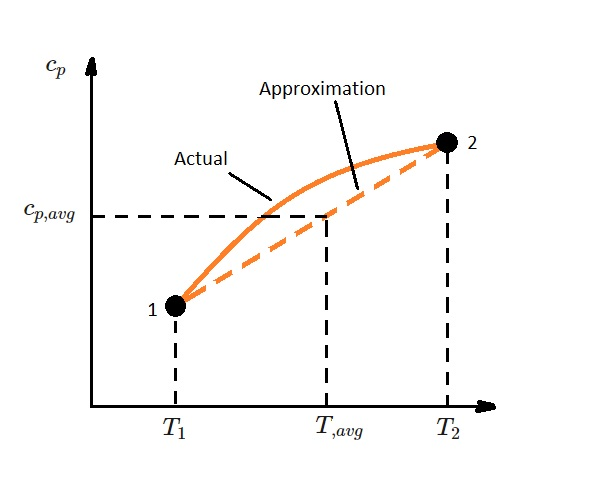# Ideal Gases: Internal Energy, and Enthalpy

Commonly the ideal gas is defined by $Pν=RT$.  In this equation $P$ is the absolute pressure, $ν$ is specific volume, $R$ is the gas constant, and $T$ is the absolute temperature.  However, internal energy and enthalpy can also be related to the ideal gas law.

### Internal Energy Ideal Gas

First, let’s discuss internal energy. To determine the internal energy of an ideal gas you would use the following equation.

(Eq 1) $u=u(T)$

Equation one shows that the internal energy of an ideal gas is a function of the temperature only.  Joule showed this through experimentation.  He did this by submerging two tanks in water that were connected with a pipe and valve.  One tank contained air at a high pressure. The other was completely evacuated.  After thermal equilibrium was obtained, the valve was opened to allow air to to pass from the high pressure tank into the evacuated tank.  During this process he noticed that the temperature of water bath did not change.  As a result, he reasoned that the internal energy is only a function of temperature and is not effected by pressure or specific volume.   However, this is only true for ideal gases.  For a real gas that differs significantly from an ideal gas, internal energy is not a function of internal energy alone.

### Enthalpy  Ideal Gas

Enthalpy is a thermodynamic  property that represents the systems internal energy plus the product of the systems pressure and volume.   In other words, it represents the total heat content of a system.  For an ideal gas, the definition of enthalpy and the equation of state is as follows.

(Eq 2) $h=u+RT=\cases{h=u+Pν \cr Pν=RT}$

However, since $R$ is constant, and $u=u(T)$, for an ideal gas enthalpy will also be a function of temperature only.

(Eq 3) $h=h(T)$

### Specific Heat Ideal Gas

Due to the fact that both enthalpy and internal energy are solely dependent on temperature, the specific heat of an ideal gas is also only temperature dependent.  As a result, the the internal energy and enthalpy of an ideal gas can be expressed using the following.

(Eq 4) $du=c_v(T)dt$

$c_v$ = specific heat at a constant volume

(Eq 5) $dh=c_p(T)dT$

$c_p$ = specific heat at a constant pressure

In order to determine the change in internal energy or enthalpy for a process you would integrate equations 4 and 5.

(Eq 6) $Δu=u_2-u_1=\int{}^2_1~c_v(T)dt$ (kJ/kg)

and

(Eq 7) $Δh=h_2-h_1=\int{}^2_1~c_p(T)dt$ (kJ/kg)

To solve these equations we will need to find a relation for $c_v$ and $c_p$ as a function of temperature.  To do this you can use data that is based off of direct measurement or calculations from statistical  behavior of molecules.  Below is a table showing the specific heat of air at various temperatures.

 Air Temperature (K) $c_p$ (kJ/kg-K) $c_v$ (kJ/kg-K) k 250 1.003 0.716 1.401 300 1.005 0.718 1.400 350 1.008 0.721 1.398 400 1.013 0.726 1.395 450 1.020 0.733 1.391 500 1.029 0.742 1.387 550 1.040 .753 1.381 600 1.051 .764 1.376 650 1.063 .776 1.370 700 1.075 .788 1.364 750 1.087 0.800 1.359 800 1.099 0.812 1.354 900 1.121 0.834 1.344 1000 1.142 0.855 1.336

In addition, over small temperatures changes, such as a few hundred degrees or less, the specific heat can be approximated as linear.  As a result, the specific heat function in equations 6 and 7 can be replaced with a constant average.  This would yield the following equations.

(Eq 8) $u_2-u_1=c_{v,avg}(T_2-T_1)$

(Eq 9) $h_2-h_1=c_{p,avg}(T_2-T_1)$### Specific Heat Relations for an Ideal Gas

For an ideal gas there is a special relationship between $c_p$ and $c_v$.  This is found by differentiating the relation $h=u+RT$.  In turn, this will yield $dh=du+Rdt$.  Next, $dh$ is replaced with $cpdT$ and $du$ is replaced with $c_vdT$.  Then after dividing the resulting equation by $dT$ we will obtain the following equation.

(Eq 10) $c_p=c_v+R$

This equation will give us the ability to determine $c_v$ when we have knowledge of $c_p$ and the gas constant $R$.  In turn, we can now introduce another ideal-gas property called the specific heat ratio $k$.  The specific heat ratio is defined using the following equation.

(Eq 11) $k=\frac{c_p}{c_v}$